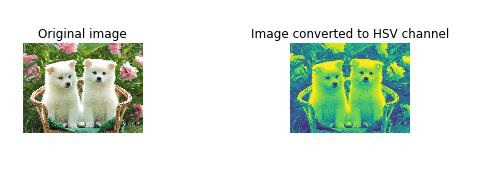# How can RGB color space be converted to a different color space in Python?

Conversion of an image from one color space to another is usually used so that the newly achieved color space can prove as a better input to perform other operations on it. This includes separating hues, luminosity, saturation levels, and so on.

When an image is represented using RGB representation, the hue and luminosity attributes are shown as a linear combination of channels R, G and B.

When an image is representing using HSV representation (here, H represents Hue and V represents Value), RGB is considered as a single channel.

Here’s the example to convert RGB color space to HSV −

## Example

import matplotlib.pyplot as plt
from skimage import data
from skimage.color import rgb2hsv
path = "path to puppy_1.JPG"
rgb_img = img
hsv_img = rgb2hsv(rgb_img)
value_img = hsv_img[:, :, 2]
fig, (ax0, ax1) = plt.subplots(ncols=2, figsize=(8, 2))
ax0.imshow(rgb_img)
ax0.set_title("Original image")
ax0.axis('off')
ax1.imshow(value_img)
ax1.set_title("Image converted to HSV channel")
ax1.axis('off')
fig.tight_layout()

## Output## Explanation

• The required libraries are imported.
• The path where the image is stored is defined.
• The ‘imread’ function is used to visit the path and read the image.
• The ‘imshow’ function is used to display the image on the console.
• The function ‘rgb2hsv’ is used to convert the image from RGB color space to HSV color space.
• The matplotlib library is used to plot this data, and show the original image and the image after being converted to HSV color space.
• This is displayed on the console.